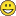# QlikView Creating Analytics

Discussion Board for collaboration related to Creating Analytics for QlikView.

HighlightedNot applicable

## To Calculate Sum of Rows with aggregated expression

I want to calculate the Total sum by Región of [PDM X Región 2] Field

I have this:

[PDM x Región 2] = [PDM x Cemento] * [Volumen %]

And I want to get the total sum = 2.47My [PDM x Región 2] Expression is: [PDM X Cemento]* Sum(Volumen)/Sum( DISTINCT TOTAL <Región> Volumen)

I've tried using the sum(aggr(sum [PDM x Región 2] , Región, Gerencia)) and doesn't work

Can anyone help me, please

Thanks a lot

BR

Tags (5)
1 Solution

Accepted SolutionsMVP

## Re: To Calculate Sum of Rows with aggregated expression

Hi BR,

if [PDM X Cemento] is a field name, try

sum(aggr( [PDM X Cemento]* Sum(Volumen)/Sum( DISTINCT TOTAL <Región> Volumen), Región, Gerencia))

2 RepliesMVP

## Re: To Calculate Sum of Rows with aggregated expression

Hi BR,

if [PDM X Cemento] is a field name, try

sum(aggr( [PDM X Cemento]* Sum(Volumen)/Sum( DISTINCT TOTAL <Región> Volumen), Región, Gerencia))Not applicable

## Re: To Calculate Sum of Rows with aggregated expression

You R a Genius!!! Thanx